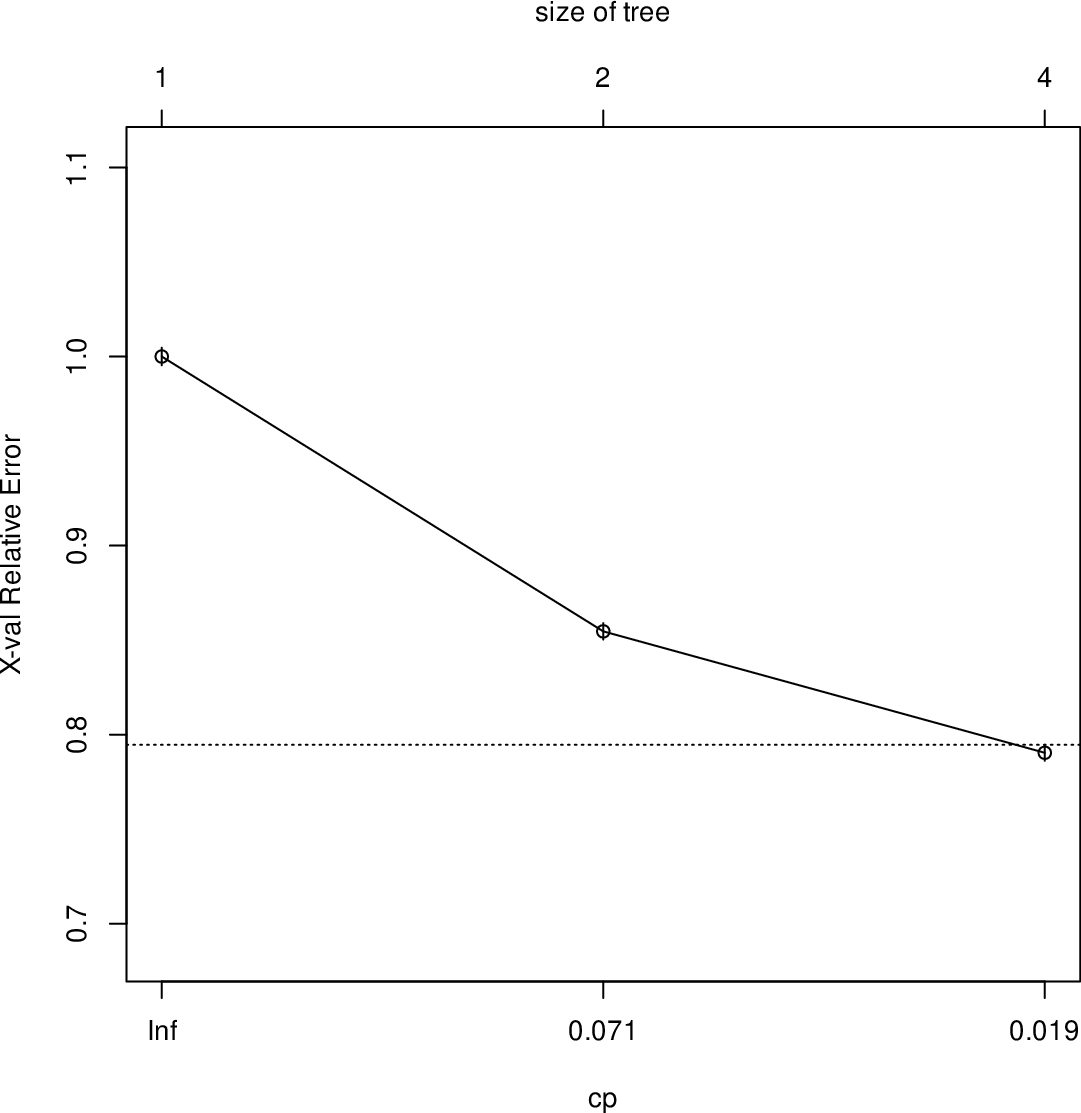Data Science Desktop Survival Guide by Graham WilliamsDesktop Survival Project Home Preface Data Science Introducing R R Constructs R Tasks R Strings R Read, Write, and Create Data Template Data Exploration Data Wrangling Data Visualisation Statistics ML Template ML Scenarios ML Activities ML Applications ML Algorithms Cluster Analysis Decision Trees Computer Vision Graph Data Privacy Literate Data Science Coding with Style Resources Bibliography Index

## Complexity Parameter Plot

The plotcp() plots the cross-validation results. Here we see a set of possible cost-complexity prunings of the tree. We might choose to prune using the leftmost complexity parameter which has a mean below the horizontal line.

 plotcp(model)